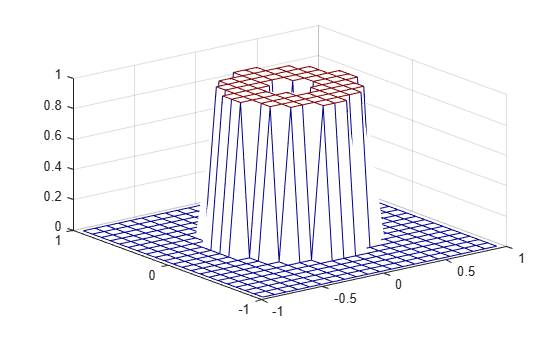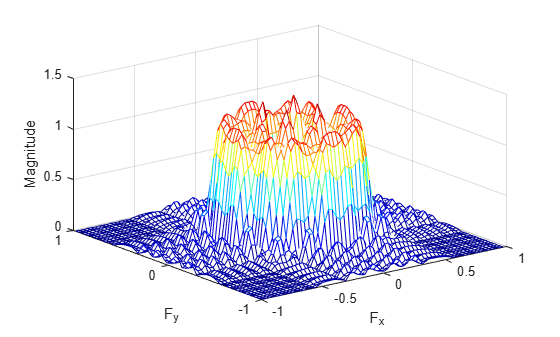fsamp2

2-D FIR filter using frequency sampling

Description

example

h = fsamp2(Hd) designs a two-dimensional FIR filter with frequency response Hd, and returns the filter coefficients in matrix h. The filter h has a frequency response that passes through points in Hd. fsamp2 designs two-dimensional FIR filters based on a desired two-dimensional frequency response sampled at points on the Cartesian plane.

h = fsamp2(f1,f2,Hd,[m n]) produces an m-by-n FIR filter by matching the filter response at the points in the vectors f1 and f2. The frequency vectors f1 and f2 are in normalized frequency, where 1.0 corresponds to half the sampling frequency, or π radians. The resulting filter fits the desired response as closely as possible in the least squares sense. For best results, there must be at least m*n desired frequency points. fsamp2 issues a warning if you specify fewer than m*n points.

Examples

collapse all

Use fsamp2 to design an approximately symmetric, two-dimensional bandpass filter with passband between 0.1 and 0.5 (normalized frequency, where 1.0 corresponds to half the sampling frequency, or $\pi$ radians).

Create a matrix Hd that contains the desired bandpass response. Use freqspace to create the frequency vectors f1 and f2.

[f1,f2] = freqspace(21,'meshgrid');
Hd = ones(21);
r = sqrt(f1.^2 + f2.^2);
Hd((r<0.1)|(r>0.5)) = 0;
colormap(jet(64))
mesh(f1,f2,Hd)Design the filter that passes through this response.

h = fsamp2(Hd);
freqz2(h)Input Arguments

collapse all

Frequency response, specified as a numeric matrix. Hd is a matrix containing the desired frequency response sampled at equally spaced points between -1.0 and 1.0 along the x and y frequency axes. The value 1.0 corresponds to half the sampling frequency, or π radians.

${H}_{d}\left({f}_{1},{f}_{2}\right)={{H}_{d}\left({\omega }_{1},{\omega }_{2}\right)|}_{{\omega }_{1}=\pi {f}_{1},{\omega }_{2}=\pi {f}_{1}}$

For best results, use frequency points returned by freqspace to create Hd.

Data Types: single | double | int8 | int16 | int32 | int64 | uint8 | uint16 | uint32 | uint64

Frequency vector, specified as a numeric vector.

Data Types: double

Frequency vector, specified as a numeric vector.

Data Types: double

Size of output FIR filter h, specified as a 2-element vector of positive integers. The filter has m rows and n columns.

Data Types: double

Output Arguments

collapse all

2-D FIR filter, returned as a numeric array. fsamp2 returns h as a computational molecule, which is the appropriate form to use with filter2. If you specify a frequency response matrix Hd, then h has the same size. If Hd is of class single, h is also of class single. Otherwise, h is of class double.

Data Types: single | double

Algorithms

fsamp2 computes the filter h by taking the inverse discrete Fourier transform of the desired frequency response. If the desired frequency response is real and symmetric (zero phase), the resulting filter is also zero phase.

 Lim, Jae S., Two-Dimensional Signal and Image Processing, Englewood Cliffs, NJ, Prentice Hall, 1990, pp. 213-217.